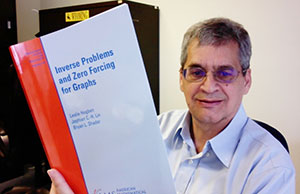apply now

# UW’s Shader Co-Author of New Mathematics Research Book That Explores Inverse Problems

September 16, 2022Bryan Shader, a professor in UW’s Department of Mathematics and Statistics, is co-author of a research monograph titled “Inverse Problems and Zero Forcing for Graphs.” The book, published by the American Mathematical Society, is geared toward graduate students and researchers interested in inverse eigenvalue problems for graphs. (Bryan Shader Photo)

Whether it relates to measuring vibrations in the architectural design of a skyscraper or the frequency and amplitude of a piano string, most inverse problems become mathematical puzzles to solve.

To provide a better understanding of inverse mathematical problems, Bryan Shader co-wrote “Inverse Problems and Zero Forcing for Graphs,” a research monograph that describes and develops new mathematical techniques in this area.

Shader, a professor in the University of Wyoming’s Department of Mathematics and Statistics, wrote the book with Leslie Hogben, a professor of mathematics at Iowa State University; and Jephian Lin, an associate professor of mathematics at National Sun Yat-Sen University in Kaohsiung, Taiwan.

The book, published by the American Mathematical Society, is geared toward graduate students and researchers interested in inverse eigenvalue problems for graphs (IEP-G). According to the book summary, the IEP-G asks a fundamental mathematical question expressed in terms of linear algebra and graph theory. However, the significance of such questions goes beyond these two areas, as particular instances of the IEP-G also appear as major research problems in other fields of mathematics, science and engineering.

“The book presents, in a unified way, the major breakthroughs in this area since 2005,” says Shader, who currently serves as interim head of the UW Department of Electrical Engineering and Computer Science. “It lays the foundation for a new area of mathematical research.”

Forward problems use mathematical invariants of models to study various phenomena, such as vibrations of a skyscraper caused by an earthquake. Inverse problems are inherently engineering problems. Rather than studying a given system, one asks if you can adjust parameters -- strengths of beams and pillars -- to achieve certain properties: in this case, a skyscraper that can withstand the vibrations caused by an earthquake, Shader says.

“An inverse problem asks, ‘Can you design a drumhead or a skyscraper so that it has certain fundamental frequencies?,’” Shader says. “How might you design a skyscraper to minimize vibrations on the upper floors for typical wind patterns or seismic activity?

“Vibrations of objects play a critical role in various applications ranging from music -- where the frequency and amplitude of a piano string’s movement are related to the note and volume that we hear -- to architecture, where large pendulums are suspended in the middle of skyscrapers to minimize the vibrations of the building due to wind, ground transportation and seismic activity,” Shader explains.

He adds that most inverse problems become mathematical problems that are about a square array of numbers -- also known as a matrix -- with a certain structure (the location of the nonzero entries fits a pattern) informed by the vibrating object and certain invariants of the array, known as eigenvalues.

Over the past 15 years, Shader and his co-authors have organized numerous international meetings on this topic.

“Several of my Ph.D. students developed techniques that truly transformed the area. It is wonderful to be able to highlight their work,” Shader says. “Two years ago, we were asked to run a mathematical research community by the American Mathematical Society. The communities are designed to expose fresh Ph.D. students to emerging areas of mathematical research. At that time, my co-authors and I decided to write the book.”Accreditation | Virtual Tour | Emergency Preparedness | Employment at UW | Privacy Policy | Harassment & Discrimination | Accessibility